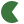{{ soundUploadQueueCount }} sounds queued for upload and conversion5

# Maximize and Minimize SoundsThe weak duality theorem states that the objective value of the dual LP at any feasible solution is always a bound on the objective of the primal LP. If the primal has an optimal solution then the dual has an optimum solution too, and the two optima are equal.
See also: Maximization, Profit maximization, Design optimization, Expectation–maximization algorithm, Mathematical optimization, and Marlett.